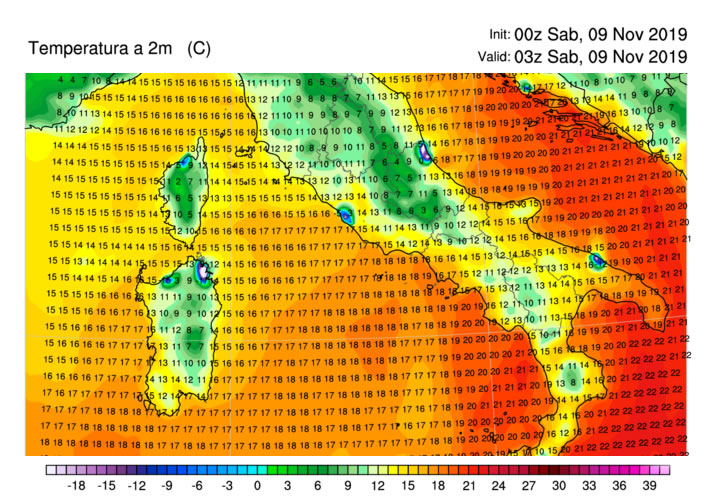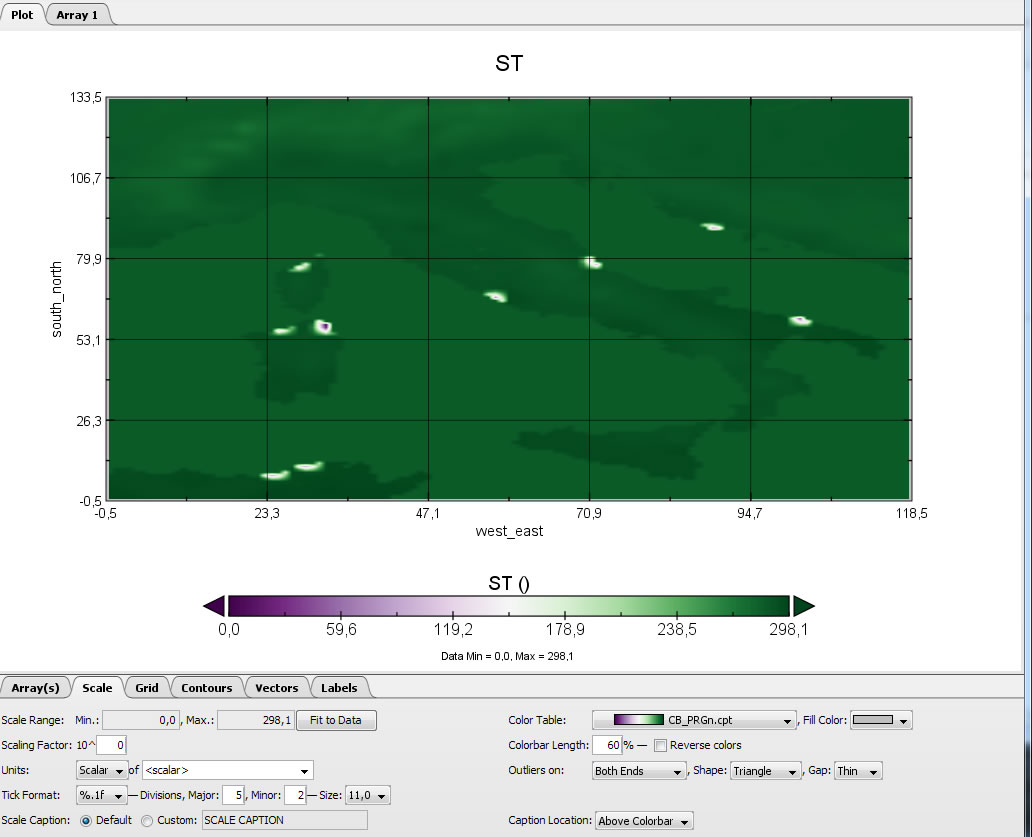## Strange extreme low temperature points

Questions and other topics related to UEMS 18.
Stefano90
Posts: 4
Joined: Sat Jan 02, 2016 10:19 am

### Strange extreme low temperature points

Hi all,
In the last days I have a strange problem with some particular points where temperatures (and all connected 2m values) are extremely low.
Furthermore, to make it work without the classic "segmentation fault" error I had to change the "sf_surface_physics" parameter from 2 (Noah) to 1.
Now wrf arrives to the end of the calculation but with these errors.Does anyone have any ideas?
Yhank you!

Posts: 1603
Joined: Wed Aug 19, 2009 10:05 am

### Re: Strange extreme low temperature points

Well yes, revert back to NOAH or NOAH-MP and fix source of segfault which is in most cases too large time-step but not always. You might want to post contents of your namelist.input file if you want more suggestion about possible causes of crashes.

Stefano90
Posts: 4
Joined: Sat Jan 02, 2016 10:19 am

### Re: Strange extreme low temperature points

Hi, thank you for your response.
Recently I didn't change anything in my namelist, but from run of 07Nov, 12Z , wrf process give the classic "segmentation error" after about 10 or 20 minutes of simulation (for example crash at 00:20 in 00Z run). I tried to set debug option to "1000" and I saw that the segmentation error occour after the call of RRTM. There are two solutions to avoid blocking the process:
1. change "ra_lw_physics" and "ra_sw_physics" from 1 to 0
2. change "sf_surface_physics" from 2 (Noah) to 1.
In both cases I have these strange surface values in some points.

This is my namelist file

Code: Select all

`````` &time_control
run_days                            = 0,
run_hours                           = 168,
run_minutes                         = 0,
run_seconds                         = 0,
start_year                          = 2019, 2019, 2019, 2011, 2011,
start_month                         = 11,   11,   11,   11,   11,
start_day                           = 10,   10,   10,   21,   21,
start_hour                          = 12,   15,   15,   06,
start_minute                        = 00,   00,   00,   00,   00,
start_second                        = 00,   00,   00,   00,   00,
end_year                            = 2019, 2019, 2019, 2011, 2011,
end_month                           = 11,   11,   11,   11,   11,
end_day                             = 17,   17,   17,   22,   22,
end_hour                            = 12,   12,   12,   12,   21,
end_minute                          = 00,   00,   00,   00,   00,
end_second                          = 00,   00,   00,   00,   00,
interval_seconds           = 10800
input_from_file            = .true.
history_interval           = 180
frames_per_outfile         = 1000
restart                    = .false.
restart_interval           = 11520
io_form_history                     = 2
io_form_restart                     = 2
io_form_input                       = 2
io_form_boundary                    = 2
debug_level                         = 0
/

&domains
time_step                  = 30
time_step_fract_num        = 0
time_step_fract_den        = 10
max_dom                    = 1
s_we                       = 1
e_we                       = 120
s_sn                       = 1
e_sn                       = 135
s_vert                     = 1
e_vert                              = 30
eta_levels                          = 1.000, 0.995, 0.990, 0.985, 0.980,
0.970, 0.960, 0.950, 0.940, 0.930,
0.920, 0.900, 0.880, 0.860,
0.830, 0.800, 0.770, 0.740, 0.710,
0.680, 0.620, 0.560,
0.460, 0.360,
0.260, 0.200, 0.140,
0.080, 0.040, 0.000,
dx                         = 9000.0000
dy                         = 9000.0000
grid_id                    = 1
parent_id                  = 1
i_parent_start             = 1
j_parent_start             = 1
parent_grid_ratio          = 1
parent_time_step_ratio     = 1
feedback                   = 0
smooth_option              = 1
grid_allowed               = .true.
max_dz                     = 1000.
numtiles                   = 1
nproc_x                    = -1
nproc_y                    = -1
num_metgrid_soil_levels    = 4
num_metgrid_levels         = 34
interp_type                = 2
extrap_type                = 2
t_extrap_type              = 2
use_levels_below_ground    = .true.
use_surface                = .true.
lagrange_order             = 1
zap_close_levels           = 500
lowest_lev_from_sfc        = .false.
force_sfc_in_vinterp       = 1
sfcp_to_sfcp               = .false.
smooth_cg_topo             = .false.
use_tavg_for_tsk           = .false.
p_top_requested            = 5000
step_to_output_time        = .true.
target_cfl                 = 1.2, 1.2
max_step_increase_pct      = 49
starting_time_step         = 12
max_time_step              = 40
min_time_step              = 12
max_ts_locs			     = 0
max_ts_level			     = 0
/

&physics
mp_physics                 = 8
cu_physics                 = 2
kfeta_trigger              = 2
cudt                       = 1
sf_sfclay_physics          = 5
sf_surface_physics         = 1
num_soil_layers            = 4
num_land_cat               = 28
num_soil_cat               = 16
bl_pbl_physics             = 5
topo_wind                  = 1
bldt                       = 0
ra_lw_physics              = 1
ra_sw_physics              = 1
isfflx                              = 1
ifsnow                              = 1
icloud                              = 1
surface_input_source                = 1
sst_skin                   = 1
sf_urban_physics                    = 1
/

&dynamics
rk_ord                     = 3
w_damping                  = 1
diff_opt                   = 1
km_opt                     = 4
diff_6th_opt               = 2
diff_6th_factor            = 0.12
base_temp                  = 290.
damp_opt                   = 3
zdamp                      = 10000.
dampcoef                   = 0.2
khdif                      = 0
kvdif                      = 0
smdiv                      = 0.1
emdiv                      = 0.01
epssm                      = 0.1
non_hydrostatic            = .true.
gwd_opt                    = 1
/

&bdy_control
spec_bdy_width             = 5
spec_zone                  = 1
relax_zone                 = 4
specified                  = .true.
nested                     = .false.
/

&namelist_quilt
nio_groups                 = 1
/
``````

Posts: 1603
Joined: Wed Aug 19, 2009 10:05 am

### Re: Strange extreme low temperature points

Stefano,

I see lot of namelist entries that are less than standard choices, and might be a cause of trouble depending on case to case scenario. I will comment on what I see as potential point where you might look for changes that might or might not help but you can try to adjust one by one and see what happens (with LSM=2 or 4).

1) 30 vertical levels is almost guaranteed that can cause trouble. I would use at least 40.
2) You don't have to specify levels manually, real.exe will do that happily for you using some standard spacing algorithms, depending on version (default algorithm is slightly different in 3.9 vs 4.0+ --> see "auto_levels_opt" entry in 4.0+ for details)
3) You are using adaptive time step with target_cfl=1.2 which is in my opinion too large (although default!). Try 1.0 instead. It will be somewhat slower but more stable, although your max_time_step is very low for grid dx (=40) so this might just change nothing much.
4) To add speed you can increase max_time_step up to ~10 times dx which is aroung 90 but this might degrade stability.
5) cudt should be = 0 for all cu_physics except KF schemes.
6) radt might be little bit too large, try 10 minutes but I don't see general problem with 20
7) slope_rad and topo_shading won't do anything at 9km dx you can just turn it off with same results
8) diff_6th_opt I recommend you do not use it (=0)
9) epssm is usually first thing to try if model crashes with sane time step values. 0.1 is default but much more stable are higher values (try 0.5 or 1.0)
10) gwd_opt I found to crash many runs. Try to not use it if there is no huge reason to turn it on.

That's it, good luckStefano90
Posts: 4
Joined: Sat Jan 02, 2016 10:19 am

### Re: Strange extreme low temperature points

Thank you,
I tried your solution without success.

Checking better I realized something very important, it seems that the error starts in the metgrid.exe process. This is what I see when I open a metgrid-generated netcdf, and here is the log. I think that something is wrong ...

http://188.138.1.107/file/metgrid.logPosts: 1603
Joined: Wed Aug 19, 2009 10:05 am

### Re: Strange extreme low temperature points

OK that change a lot of things.

Look at geo_em.d01.nc file and see if there are any irregularities around those anomaly areas. Looks like some problems with landmask. See this if helps:
viewtopic.php?f=9&t=1066

Stefano90
Posts: 4
Joined: Sat Jan 02, 2016 10:19 am

### Re: Strange extreme low temperature points

Great, that is the solution!

Thank you very much for your advices